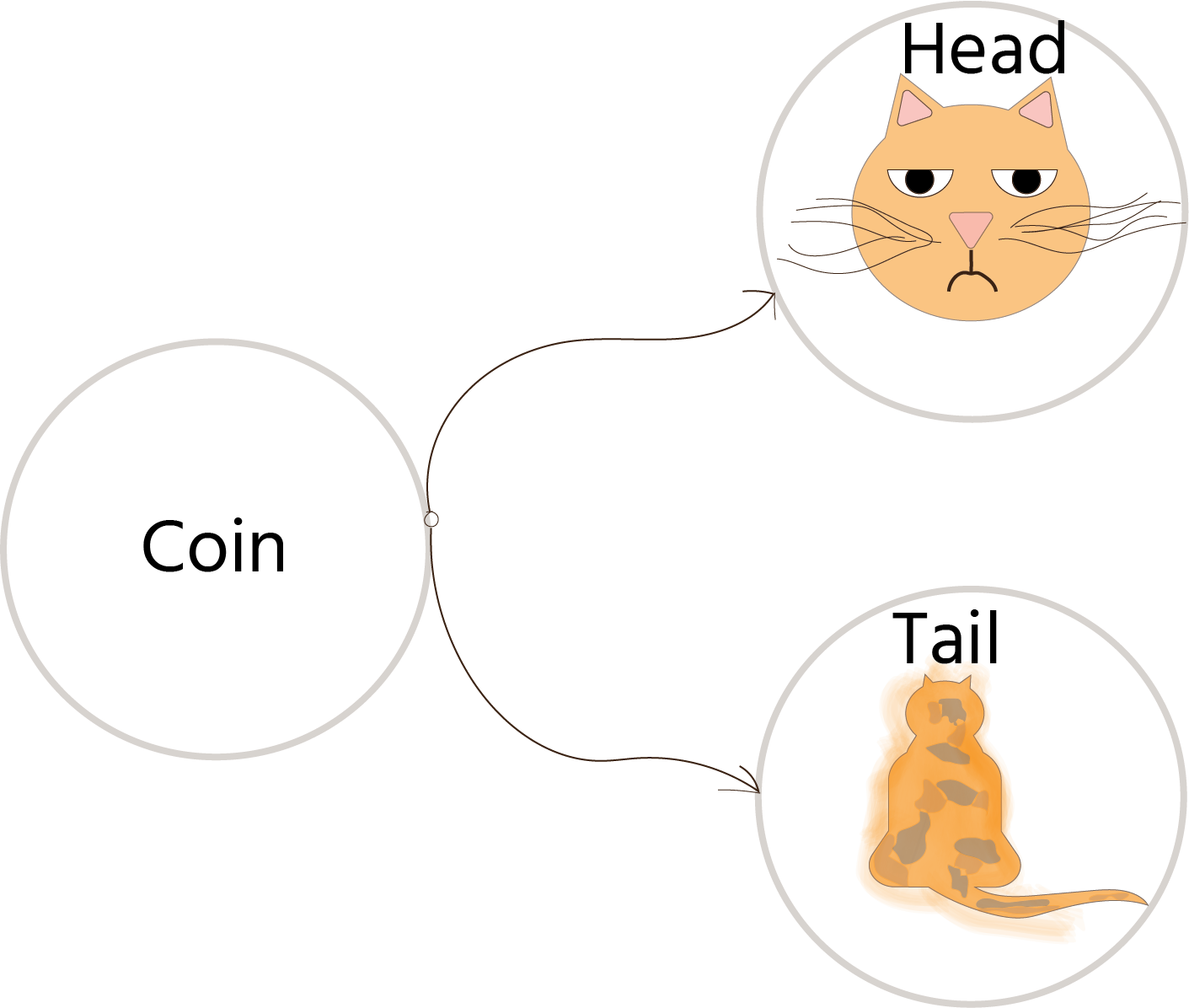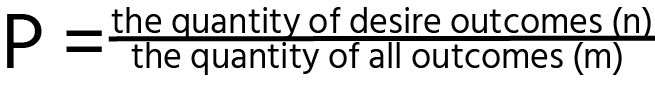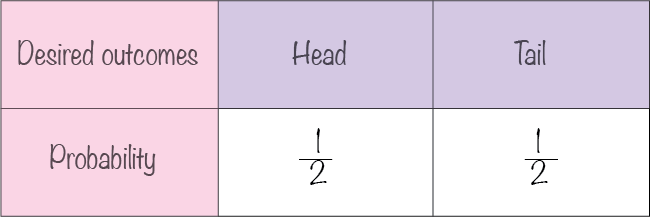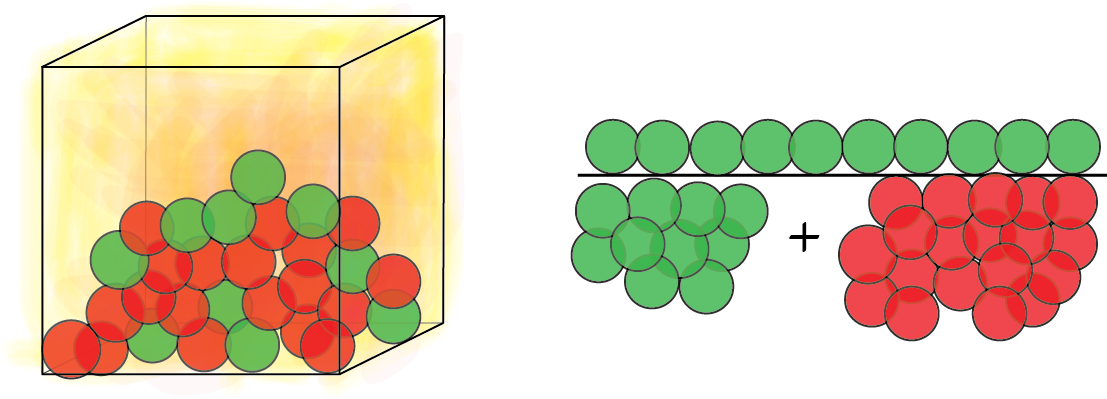Course Content

Probability TheoryRandom Variable

Have you ever wondered how often you usually use the word probability? It is time to dive deeper into this very familiar term!

Please take a look at the simplest example: imagine that you toss a coin, it is always a 50% chance of getting head or tail. I mean that there are only two possible outcomes, and the probability for each case is 1/2 (1/2 equal to 50 %).

It is time to turn to the definition of the random variable and clarify how we received such numbers.

The random variable is a quantity that equals one and only one value depending on the result of testing result.

Let's assume that X is the random variable with several outcomes (getting head or tail); therefore, we can mark them as x1 and x2.The formula for calculating the probability is the following:Let's build a small table for the probability distribution of random variables:

I hope you're interested in learning probability theory now! Here, it would be best to memorize the formula written above and the definition of a random variable.Indeed, each event can result either in success or failure. For instance, if you have a box with balls colored differently (red or green) and want to put a red one, your event has two outcomes: red ball or not a red ball.#Calculate the probability of getting a green ball out of the box with green and red balls. Look at the definition of probability and divide the amount of desired events by the quantity of all events. Here you have 10 green balls and 19 red balls.
`probability = ``/`